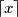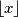### IMO Shortlist 1998 problem C1

Kvaliteta:
Avg: 0,0
Težina:
Avg: 6,0
A rectangular array of numbers is given. In each row and each column, the sum of all numbers is an integer. Prove that each nonintegral number$x$ in the array can be changed into either$\lceil x\rceil$ or$\lfloor x\rfloor$ so that the row-sums and column-sums remain unchanged. (Note that$\lceil x\rceil$ is the least integer greater than or equal to$x$, while$\lfloor x\rfloor$ is the greatest integer less than or equal to$x$.)
Izvor: Međunarodna matematička olimpijada, shortlist 1998